472kHz.org

### Weak signal modes

#### Forward error correction (FEC)

Error correction techniques
QRSS and DFCW are analog modes where reduction of the bandwidth is used to improve SNR. Another route to improve SNR is to go digital and use a error correction method. The 2 main error correction methods are:

• CRC (Cyclic Redundancy Check): with this method the transmitted data is split into packets. To each packet some additional bits, called the checksum, are added. This checksum can be used to verify if the packet is received without error. If this is not the case a request to repeat the package is send back.
A simple example: assume we split the transmitted data in 10 bit packets, thus each packet can have a value between 0 and 1023 (210-1). Let's say our packet is '1010010110', decimal 662. Now we divide this value by 8 and take the reminder. In this case the integer devision 662/8=82, the reminder is 6 (662-8·82) or binary '110'. This reminder is added to the packet, that becomes ''1010010110110'. The checksum (last 3 bits) can be used to check if the data (first 10 bits) is received well.
CRC is used in the Packet radio (AX25 protocol). Note that CRC requires a 2-way communication.
• FEC (Forward Error Correction): with this technique we do not transmit the message itself, but a much longer 'code'. This longer code allows error correction at the RX side, without the need of backward communication.
The most straightforward FEC method is the repetition code, where each bit of the message is repeated a number of times. This FEC technique is very intuitive and is also spontaneously used in phone and CW: if conditions are poor or QRM is high we tend do repeat a piece of information (eg. report) several times to ensure that it is copied correctly. But there are far more efficient FEC methods.
FEC is used in several modes that are popular on 630 m, such as WSPR, JT9 and Opera.

There are many so called FEC coding schemes, but the basic principles are the same for all. An excellent article that demonstrates and explains the fundamental principles behind Forward Error Correction codes, without diving into math, is 'The principle of forward error correction' by Paul Nicholson.

Redundancy
As seen before in 'Dot length and SNR', SNR can be improved by slowing down the transmission rate. Assume a 10 bit message is send at a rate of 1 second per bit (thus the message takes 10 seconds). If the message is sent at a slower rate of 10 seconds per bit (the message now takes 100 seconds) SNR will improve by 10 dB.
The picture below shows a simulation of the correct detections versus SNR of a 10 bit message at 1 bit per second and 1 bit per 10 seconds.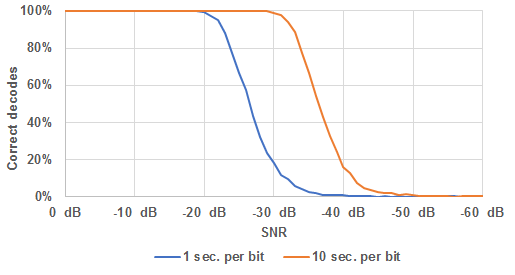As expected the SNR of the 10 seconds per bit message is about 10 dB better, with a 90% correct detection threshold at -32.7 dB versus -22.6 dB for the 1 second per bit message.
Can we do better using FEC? Instead of multiplying the bit length by 10, the bit rate is left unchanged but the 10 bits are replaced by a series of 100 bits. To avoid confusion this series of 100 bits is called a code (to distinguish it from the 10 bit message) and the 'bits' of the code are called symbols. So we have a message composed of 10 bits that is replaced by a code composed of 100 symbols.
As each bit of the message takes 10 seconds and each symbol of the code only 1 second, both the 10 bit message and the 100 symbol code will take 100 seconds to be transmitted.
Replacing the short message by a long code seems a very odd thing to do, as can be expected that the 100 symbol code will produce less correct detections than a 10 bit message (at the same bit/symbol rate): the chance to get (at least) one bit or symbol wrong will increase the more bits/symbols are transmitted and the faster they are transmitted. And indeed, the simulation show below shows that it is so!Once the errors start to occur (at about -18 dB SNR) the 100 bit message takes a much steeper dive than the 10 bit message. But fortunately there is more involved in FEC than just sending long codes instead of short messages.
For a 10 bit message there are 1024 (210) different possibilities, and each of this is expanded to a 100 symbol code. But for the 100 symbol code there are 2100 possibilities, this is over a nonillion (1 followed by thirty 0's). But only 1024 of this nonillion codes are valid, meaning they correspond to 10 bit message.
Converting the message into a code (at the TX side) is called 'coding'. At the RX side we must distinguish between 2 processes: on one hand 'detection' where the incoming (noisy) signal is converted back into a digital signal (the code) and on the other hand 'decoding' where the message is retrieved from the code.
Due to the extreme low 'valid code ratio' we can be very sure that, if a received 100 symbol code matches a valid code, the message is copied correct. There is almost no room for lucky shots. Even if the received code differs just a few symbols from a valid code there is a very high chance that this valid code was transmitted. So if instead of looking for an exact match, decoding is now done by looking which of the 1024 valid codes matches best (has the lowest number of different symbols) to the received code? To minimise the chance on a wrong decode the 1024 codes are spread over the nonillion possible 100 bit combinations in a way the mutual difference between all valid codes is as large as possible.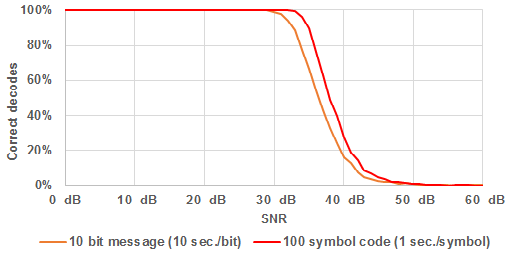If this 'best match' decoding of a 100 symbol code at 1 second per bit is compared with the detection of a 10 bit message at 10 seconds per bit (both take 100 seconds), the 100 symbol code performs about 2 dB better (90% correct decodes/detections threshold of  -34.8 dB versus -32.7 dB).
This technique of transmitting more bits (oops ... symbols) than strictly required for the message is called redundancy and the the number of different symbols between the received code and a valid code is called the hamming distance. So from all valid codes we select the one with the lowest hamming distance to the received code.

Hard-decision versus soft-decision
Let's have a look how the detection of the signal has been done so far:For transmitted digital code a '1' symbol equals +1 V and a '0' symbol equals -1 V (picture above at the left). The code is received with some noise, in this case the noise level corresponds to a SNR of 0 dB (picture above in the centre). At this SNR the '1' and '0' symbols are still visible in the noisy signal. Detection is done by averaging the received signal for the duration of each code (picture above at the right). The result can be hardly distinguished from the original transmitted code. If the averaged signal is positive (above 0 V) it is detected as a '1', if it is negative it is detected a '0'.
What happens if the noise level is increased, in order to reduce the SNR to -20 dB?Now the symbols are completely buried in the noise. After averaging all 1's are still positive and all 0's negative, so the message is decoded correct even though for symbol 1 and 5 the outcome is very close to zero (0.09 V and -0.11 V) while 2 and 4 are close to the original values of respectively 1 V and -1 V. But as detection is done by just checking whether the averaged value is positive or negative this does not matter. This is called 'hard-decision': each received symbol is assigned a 1 or 0 value. The outcome of this detection is used to select the valid code with the lowest hamming distance.

If we look at the averaged amplitude of each symbol in the above example we can be pretty sure about the state of symbol 2 and 4, while this is much more doubtful for 1 and 5. Decoding can be further improved by not just making a 1/0 decision, but instead taking the averaged value of each symbol into account. This is called 'soft-decision'. It is assumed that the larger the absolute value the more likely that the symbol is detected correct.
A efficient soft-decision method is cross-correlation. With cross-correlation the similarity between 2 signals is measured. This technique is suited very well for finding a certain pattern in a noisy signal.
A straightforward way of cross-correlation is to multiply the received (noisy) signal with the each of the valid 100 symbol codes (where again a '1' is +1 V and a '0' is -1 V) and find out with what code the largest average value is produced. By multiplying the received signal with a symbol code the received signal is inverted during the negative ('0') symbols. So regardless of a '1' and '0', positive values indicate a match between the signal and the code while negative values indicate the opposite.
If the received signal is multiplied with the correct code the result is: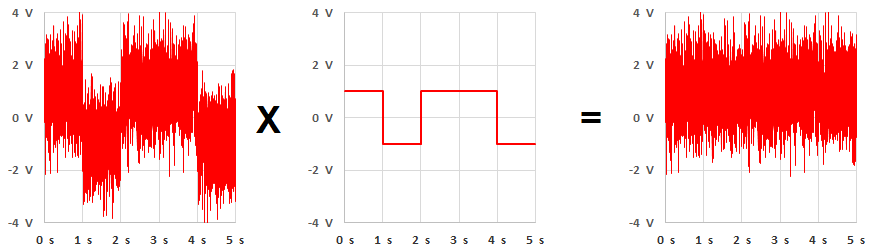If the same is done with a wrong code (the 3rd symbol is flipped) the error will show up as a negative signal after multiplication: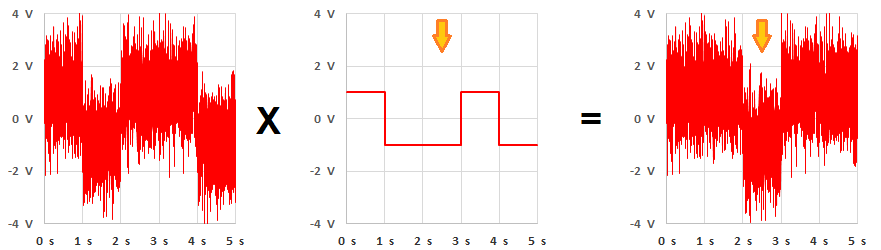Multiplication in the time domain is equivalent to mixing in the frequency domain, keeping that in mind the example below might help to understand cross-correlation:
Assume we have a very noisy signal that contains a 'message' in the way of 1000 Hz tone. One way to check the presence of this 'message' would be to mix the noisy signal with a 1000 Hz sine. Any 1000 Hz component in the noise (= the 'message') will produce a DC component at the mixer output. So if we send the mixer output signal trough a low pass filter we can retrieve this DC component. Or said otherwise: the DC component after mixing is a measure for the similarity of the noisy signal and the 1000 Hz sine.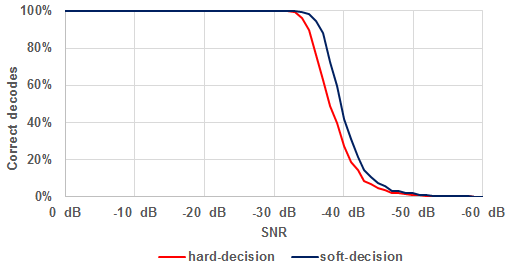As can be seen from the above picture soft-decision decoding (cross-correlation) performs about 2 dB better than hard-decision decoding (90% correct decodes threshold of -36.7 dB versus -34.8 dB).

FEC coding schemes
So far there was no particular system to generate the FEC code based on the message. Each of the 1024 (10 bit) messages was assigned a 100 symbol code in a random way, with the single constraint that the mutual difference between all valid codes is as large as possible. This means that we need to create a codebook that contains all valid codes and is known by the transmitter and the receiver. This is manageable for a 10 bit message, with 1024 valid codes in the codebook. But WSPR for example uses a 50 bit message, so there can be 250 = 1125899906842624 different messages. Creating random codes is not obvious and the size of the codebook would be gigantic: for WSPR the 50 bit message is converted to a 162 bit code, the codebook would take 182 PB (PB = petabyte, 1 PB = 1000 TB). It is more convenient to use some coding scheme to generate the codes. If this scheme is known at the TX and RX side no codebook is needed.
As mentioned in the introduction the most basic and straightforward FEC coding scheme is the repetition code, but there are more efficient coding schemes.

Convolutional code
A popular coding scheme, used in modes such as JT9 and WSPR, is the convolutional code. WSPR, for example, uses a 'non-recursive convolutional code with a constraint length of 32 and a rate of ½'. Quite a mouthful, but it sounds more complicated than it is.
A convolutional code is gradually created (encoded) from the message that has to be transmitted. Assume a 10 bit message: '1100101001'. To encode the message a part of the message is taken, called the 'window'. In this example we will take a 3 bit window and we will name the bits bA, bB and bC. The first window contains the 3 leftmost bits (MSB): '110'. Thus bA = 1, bB = 1 and bC = 0. From these 3 bits a number of 'parity bits' are derived, in this case 2 parity bits (named pA and pB):

• pA = even parity bit of bA, bB and bC (noted as bA⊕bB⊕bC)
• pB = even parity bit of bA and bC (noted as bA⊕bC)

Even parity means that the parity bit and the window bits together contain an even number of '1' bits (in fact ⊕ is the exclusive OR function). This means that the parity bit is 1 for an odd number of '1 bits' in the window and 0 for an even number. Some examples: 1⊕1⊕1 = 1, 1⊕0⊕1 = 0, 1⊕1 = 0, 0⊕1 = 1, 0⊕0 = 0, ...
In this case pA = 1⊕1⊕0 = 0 and pB = 1⊕0 = 1, thus the first 2 bits of the convolutional code are '01'.
Bits 1, 2 and 3 were used to calculated the first 2 parity bits. For the next 2 parity bits we shift the window 1 bit to the right in the source message. Now bit 2 (as bA), bit 3 (as bB) and bit 4 (as bC) are in the window: '100'. Thus the 3rd parity bit is pA = 1⊕0⊕0 = 1 and the 4th is pB = 0⊕0 = 0. These parity bits are added to the convolutional code that now becomes '0110'.
We continue to shift the window through the message until the end is reached, and for each step the parity bits are calculated and added to the convolutional code.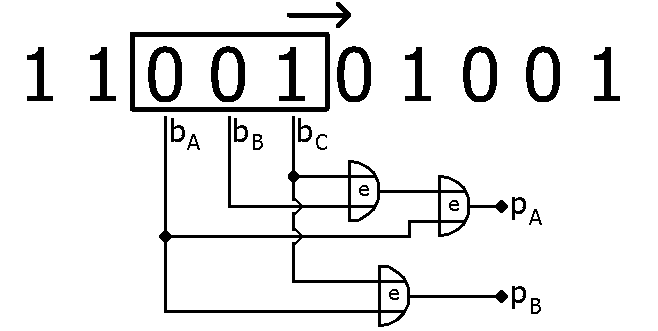The picture above shows the 3rd step with bits 3, 4 and 5 in the window. The parity bits are '11' and the convolutional code now becomes '011011'.
If we continue doing this until the window reaches the end of the message the window will do 8 steps (starting with bit 1, 2, 3 and ending with bit 8, 9, 10). For each step 2 parity bits will be generated, resulting in a convolutional code of 16 symbols. As before the notation symbols is used for elements of a code while bits is used for elements of a message.
But there is an imperfection in the coding: not all bits spend the same time in the sliding window! The bits in the centre of the message stay in the window during 3 steps, but bit 2 and 9 only during 2 steps and bit 1 and 10 even only 1 step. To fix this, W-1 (W = length of the window) 'dummy' bits (0's) are added to the start and end of the message. That way each of the 'real' bits will spend the same time in the window. In this case the message is expanded to '00110010100100'. Now the window starts with '001' and slides in 12 steps to '100'. These dummy bits will be marked red throughout the text.
If N is the number of message bits (without the dummy bits) and K is the number of bits in the window then the number of steps needed to slide the window through the entire message equals N+K-1 (in this case 10+3-1 = 12). If R is the number of parity bits generated in each step then the length of the convolutional code (dummy bits excluded) is:

C = (N+K-1)·R

In this case the length of the convolutional code is (10+3-1)·2 = 24 symbols, the code itself is '110101111110001011111011'. Note that the convolutional code only contains the parity bits, not the bits of the message.
Now let's have another look at the impressive name of the WSPR coding system: 'non-recursive convolutional code with a constraint length of 32 and a rate of ½'. The constraint length is just the number of bits in the sliding window (K) and the rate (r) is the inverse of the number of parity bits generated per step (r = 1/R). Non-recursive means that there no feedback in the calculation of the parity bits. With recursive coding parity bits are fed back in the stream of message bits and that way they will affect the value of 'future' parity bits.
Thus in our example a 'non-recursive convolutional code with a constraint length of 3 and a rate of ½' is used.

Decoding
In the previous examples decoding was done by comparing the detected signal with all the valid codes and taking the best match. For a 10 bit message (1024 valid codes) this is a feasible task. But for a 50 bit message there are over a quadrillion (1015) valid codes, checking all these codes one by one is not realistic: even if checking one code takes only 1 µs it would take over 30 years to check all valid codes. We definitely need a smarter decoding system!
A so called 'trellis decoder' is such a system. How a trellis decoder works can best be explained based on a short message that is coded with a short constraint length. Here we will use a 4 bit message '1101' that is encoded using the 'non-recursive convolutional code with a constraint length of 3 and a rate of ½' we used before. If the dummy bits are added the message is expanded to 8 bits: '00110100'. The trellis can also be used to visualize the encoding of the message:In the trellis the 2 leftmost bits of the window (bAbB) for each step in the encoding process. The first window contains '001', so we start the trellis at position '00' (step 1). The first window produces the first 2 parity bits: '11'. For the next step the window shifts one position to the right and becomes '011', thus we move to position '01' in the trellis. That way we shift the window through the entire message and note the code (parity bits) for each step. The result is '110101001011' as code.
For the decoding we can also use the trellis. In that case we start from the received code, split this into pairs of symbols ('110101001011' → '11' + '01' + '01' + '00' + '10' +'11') and use the trellis to retrieve the message. But whereas for the encoding the conversion from window to code is unambiguous (each 3 bit window can produce only one 2 symbol code pair) this is not the case for decoding: for each 2 symbol code pair there are 2 possible windows! For example, the code '10' can originate from the windows '010' or '111'. So one way or another we must be able to exclude one of the two in the decoding process.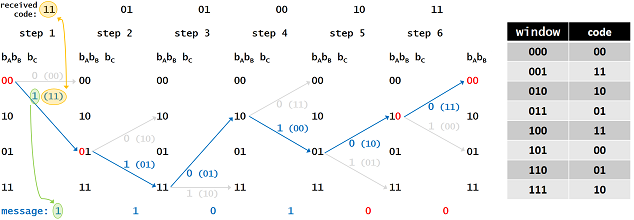To decode the received code we take the code symbols pair by pair (as they were generated and see how they lead us through the trellis. As we know that the expanded message starts and ends with the dummy bits '00' we know that we must start the trellis at the position '00' and also end the trellis at the position '00'. The above trellis shows how the decoding is done if we received the code without errors ('110101001011'). Again we split the received code into pairs of code symbols and put then above the trellis:

• Step 1: As we know that the expanded message starts with '00' the 1st window must be '000' (→ code symbol pair '00') or '001' (→ code symbol pair '11'). As the received 1st code symbol pair is '11' the window '001' fits, so the 1st message bit (apart from the leading dummy bits) must be '1'.
• Step 2: If the 1st window is '001' the 2nd window must start with '01', either '010' (→ code symbol pair '10') or '011' (→ code symbol pair '01'). As the received 2nd symbol pair is '01' the window '011' fits and thus the 2nd message bit is '1'.
• Step 3: If the 2nd window is '011' the 3rd window must start with '11', either '110' (→ code symbol pair '01') or '111' (→ code symbol pair '10'). As the received 3rd symbol pair is '01' the window '110' fits and thus the 3rd message bit is '0'.
• Step 4: If the 3rd window is '110' the 4rd window must start with '10', either '100' (→ code symbol pair '11') or '101' (→ code symbol pair '00'). As the received 4rd symbol pair is '00' the window '101' fits and thus the 4rd message bit is '1'.
• Step 5: If the 4th window is '101' the 5th window must start with '01', either '010' (→ code symbol pair '10') or '011' (→ code symbol pair '01'). As the received 5th symbol pair is '10' the window '010' fits and thus the next message bit (1st tailing dummy bit) is be '0'.
• Step 6: If the 5th window is '010', the 6th window must start with '10', either '100' (→ code symbol pair '11') or '101 (→ code symbol pair '00'). As the received 6th symbol pair is '11' the window '100' fits and this confirms that the second tailing dummy bit is indeed '0'.

The outcome of the decoding process is the correct message '1101'. Above we have reasoned each step of the decoding. A faster way is to project the 2 possible windows for each step on the trellis and look for a uninterrupted path from start to finish. This path represents the correct decoding.

What happens if the code is received incorrect, for example if 8th symbol is flipped ('110101011011' instead of '110101001011')? The first 3 symbol pairs are received correct, so step 1, 2 and 3 will be the same as above and we know that the 1st and 2nd bit are 1 and the 3rd bit is 0. But the 4rd symbol pair contains the error: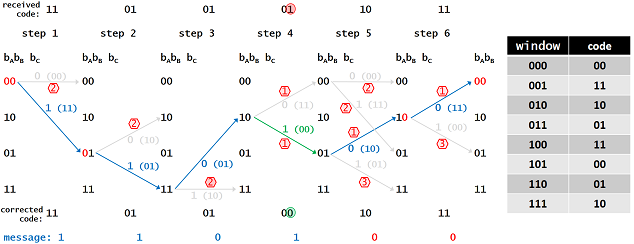• Step 4: If the 3rd window is '110' the 4rd window must start with '10', either '100' (→ code symbol pair '11') or '101' (→ code symbol pair '00'). But the received 4rd symbol pair is '01', it doesn't fit with neither '100' nor '101'. It is clear that an error occurred and we must try to fix it.
As the error showed up in step 4 we start with assuming that one of the symbols in the 4rd pair is flipped:
• If the 1st symbol is wrong (thus '11' is correct) the 4rd window is '100' and the 4rd message bit is 0.
• If the 2nd symbol is wrong (thus '00' is correct) the 4rd window is '101' and the 4rd message bit is 1.
At this moment we don't know which of both is correct, but we can figure this out in the next step.
• Step 5: The 5th code symbol pair is '10'.
• If the 4rd window is "100' the 5th window must start with '00', either '000' (→ code symbol pair '00') or '001' (→ code symbol pair '11'). None of these fit with the received '10'.
• If the 4rd window is "101' the 5th window must start with '01', either '010' (→ code symbol pair '10') or '011' (→ code symbol pair '01'). Now '010' fits and thus the 4rd bit is 1 and the 5th bit (1st tailing dummy bit) is 0.
• Step 6 is identical to the previous example and confirms that the 2nd tailing dummy bit is 0 as expected.

By changing 1 symbol in the received code we were able to create a valid path through the trellis and decoded the message as '1101'. But before concluding that this is our 'best shot' we should check if there are no other valid path that with also just 1 wrong symbol. So all possibilities should be checked until the number of errors exceeds 1:

• Step 1: Assume that the 1st window is '000' (→ code symbol pair '00'). '00' however differs 2 symbols from the received '11', so we can stop tracing that path right away.
• Step 2: Assume that the 2nd window is '010' (→ code symbol pair '10'). '10' however differs 2 symbols from the received '01', so we can stop tracing that path too.
• Step 3: Assume that the 3rd window is '111' (→ code symbol pair '10'). '10' differs again 2 symbols from the received '01', so we can stop that path either.
• Step 4: Both possible windows  '100' (→ code symbol pair '11') and '101' (→ code symbol pair '00') differ 1 symbol from the received '01'. This means that is step 5 we have to examine all 4 possibilities.
• Step 5: The 5th code symbol pair is '10'.
• If the 4rd window is "100' and the 5th window is '000' (→ code symbol pair '00'), we have 2 different symbols in total.
• If the 4rd window is "100' and the 5th window is '001' (→ code symbol pair '11'), we have again 2 different symbols in total.
• If the 4rd window is "101' and the 5th window is '011' (→ code symbol pair '01'), we have 3 different symbols in total.
• Step 6: As the previously examined path (blue path in the trellis) contains no errors it is not necessary to check alternative paths.

With one wrong symbol in the code the message is still decoded correct, we were able to detect and correct the symbol error! The decoding algoritm is able to detect and correct even more than 1 symbol error. It would lead to far to investigate all possibilities, but below you find a simulator where you can see how the decoding is done, step by step, for any 12 symbol code.
The correct code (for the message '1101') is '110101001011'. The simulator shows the received code at the top, it can be changed by clicking on the code pair before decoding is started. The trellis shows the different paths that are investigated during the decoding process, the best continuous path(s) as blue lines the others as grey lines. At the end of each line the number of detected symbol errors is shown. Below the trellis the corrected code pairs and the decoded message is show. Start the decoding by clicking on 'Start' and proceed by clicking on 'Next step':

Your browser does not support HTML5 Canvas

Interleaving and synchronisation
Convolutional coding is pretty robust in regard with erroneous symbols as long as these are no too close to each other. This can cause problem in real communication as QRM and QRN bursts tend to corrupt consecutive symbols. In order to minimise the devastating effect of these bursts the code is interleaved: the sequence of the symbols is changed so the original neighbor symbols are transmitted well spaced. Of course at the RX side is known how the symbols are shuffled, so they can be restored in the correct order before decoding.
An example: Assume a 8 symbol message 's1s2s3s4s5s6s7s8'. Before transmitting we chance the symbol order to 's3s8s5s1s4s7s2s6'.

Another issue can be synchronisation. In order to detect the symbol stream it must be known when a symbol starts (and ends). With radio communication synchronisation must be retrieved from the incoming signal, using the 1-0 and 0-1 transitions of the received code. But this code often contains long series of 1 or 0 symbols and thus insufficient transitions to allow a proper synchronisation. For that reason often a pseudo random pattern is sliced into the code, inducing a sufficient number of transitions. Again at the RX side the positions of these pattern symbols is known, so they can be removed prior to decoding.

 previous content next